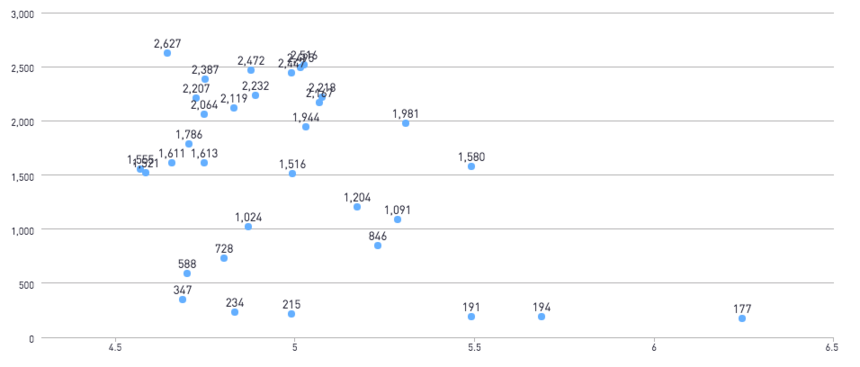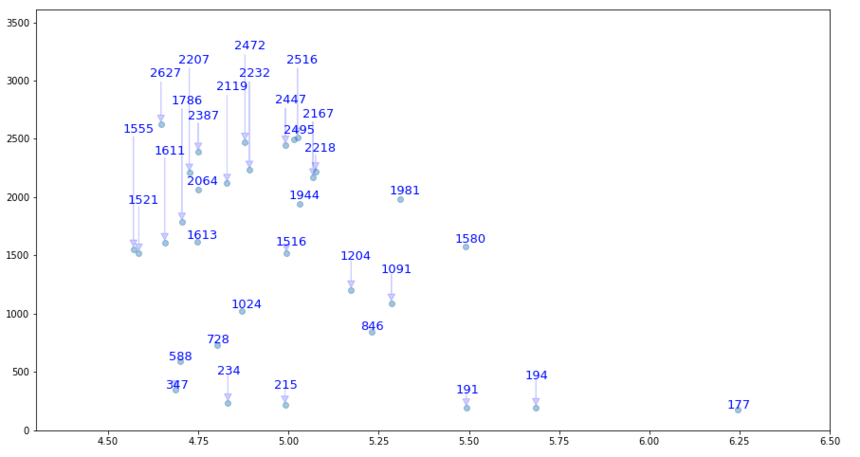Getting Around Overlapping Data Labels With Python

A common hazard when adding data labels to a chart, is that often times the data labels will overlap when there are two points located relatively close to one another. Fortunately, the flexibility of python allows us a way around overlapping data labels.

Here is my original chart as a Periscope scatter plot with data labels applied,We can see the the data labels overlap in some areas making it hard to read and visually unappealing.

Instead we can create a Python chart and write a custom function that checks the location of the data-labels and adjusts any that overlap.

import numpy as np
import matplotlib.pyplot as plt
import pandas as pd

# source: https://stackoverflow.com/questions/8850142/matplotlib-overlapping-annotations

def get_text_positions(x_data, y_data, txt_width, txt_height):
a = zip(y_data, x_data)
text_positions = y_data.copy()
for index, (y, x) in enumerate(a):
local_text_positions = [i for i in a if i > (y - txt_height)
and (abs(i - x) < txt_width * 2) and i != (y,x)]
if local_text_positions:
sorted_ltp = sorted(local_text_positions)
if abs(sorted_ltp - y) < txt_height: #True == collision
differ = np.diff(sorted_ltp, axis=0)
a[index] = (sorted_ltp[-1] + txt_height, a[index])
text_positions[index] = sorted_ltp[-1] + txt_height
for k, (j, m) in enumerate(differ):
#j is the vertical distance between words
if j > txt_height * 1.5: #if True then room to fit a word in
a[index] = (sorted_ltp[k] + txt_height, a[index])
text_positions[index] = sorted_ltp[k] + txt_height
break
return text_positions

def text_plotter(x_data, y_data, text_positions, axis,txt_width,txt_height):
for x,y,t in zip(x_data, y_data, text_positions):
axis.text(x - .03, 1.02*t, '%d'%int(y),rotation=0, color='blue', fontsize=13)
if y != t:
axis.arrow(x, t+20,0,y-t, color='blue',alpha=0.2, width=txt_width*0.0,

x_data = df['avg']
y_data = df['count']

fig = plt.figure(figsize=(15,8))
ax.scatter(x_data, y_data, alpha = 0.4)
txt_height = 0.04*(plt.ylim() - plt.ylim())
txt_width = 0.02*(plt.xlim() - plt.xlim())
text_positions = get_text_positions(x_data, y_data, txt_width, txt_height)
text_plotter(x_data, y_data, text_positions, ax, txt_width, txt_height)

plt.ylim(0,3610)
plt.xlim(4.3,6.5)

periscope.output(plt)

Here is our new python scatter plot: